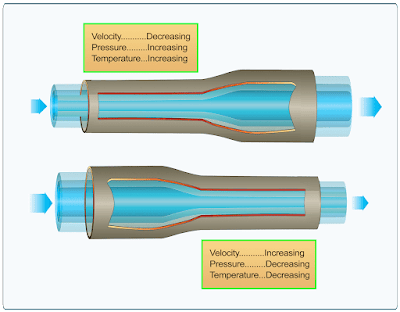# Aircraft Gas Turbine Engines Thrust

Using the formula below, compute the force necessary to accelerate a mass of 50 pounds by 100 ft/Sec2.

This illustrates that if the velocity mass per second is increased by 100, the resulting thrust is 155 pounds.

Since the turbojet engine accelerates air, the following formula can be used to determine jet thrust:

As an example, to use the formula for changing the velocity of 100 pounds of mass airflow per second from 600 ft/sec to 800 ft/sec, the formula can be applied as follows:

As shown by the formula, if the mass airflow per second and the difference in the velocity of the air from the intake to the exhaust are known, it is easy to compute the force necessary to produce the change in the velocity. Therefore, the thrust of the engine must be equal to the force required to accelerate the air mass through the engine. Then, by using the symbol “Fn” for thrust pounds, the formula becomes:

Thrust of a gas turbine engine can be increased by two methods: increasing the mass flow of air through the engine or increasing the gas velocity. If the velocity of the turbojet engine remains constant with respect to the aircraft, the thrust decreases if the speed of the aircraft is increased. This is because V1 increases in value. This does not present a serious problem, however, because as the aircraft speed increases, more air enters the engine, and jet velocity increases. The resultant net thrust is almost constant with increased airspeed.

The Brayton cycle is the name given to the thermodynamic cycle of a gas turbine engine to produce thrust. This is a variable volume constant-pressure cycle of events and is commonly called the constant-pressure cycle. A more recent term is “continuous combustion cycle.” The four continuous and constant events are intake, compression, expansion (includes power), and exhaust. These cycles are discussed as they apply to a gas-turbine engine. In the intake cycle, air enters at ambient pressure and a constant volume. It leaves the intake at an increased pressure and a decrease in volume. At the compressor section, air is received from the intake at an increased pressure, slightly above ambient, and a slight decrease in volume.

Air enters the compressor where it is compressed. It leaves the compressor with a large increase in pressure and decrease in volume, created by the mechanical action of the compressor. The next step, expansion, takes place in the combustion chamber by burning fuel, which expands the air by heating it. The pressure remains relatively constant, but a marked increase in volume takes place. The expanding gases move rearward through the turbine assembly and are converted from velocity energy to mechanical energy by the turbine. The exhaust section, which is a convergent duct, converts the expanding volume and decreasing pressure of the gases to a final high velocity. The force created inside the engine to keep this cycle continuous has an equal and opposite reaction (thrust) to move the aircraft forward.

Bernoulli’s principle (whenever a stream of any fluid has its velocity increased at a given point, the pressure of the stream at that point is less than the rest of the stream) is applied to gas turbine engines through the design of convergent and divergent air ducts. The convergent duct increases velocity and decreases pressure. The divergent duct decreases velocity and increases pressure. The convergent principle is usually used for the exhaust nozzle. The divergent principle is used in the compressor and diffuser where the air is slowing and pressurizing.When air passes through a diverging duct, air velocity decreases while air pressure and temperature increase. When air passes through a converging duct, air velocity increases while air pressure and temperature decrease

RELATED POSTS